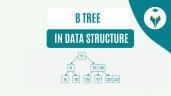Linear Search Algorithm (with Code)

# Linear Search Algorithm (with Code)

clickHere
Updated on Jul 14, 2022 10:40 IST

The below article explains linear search. Also, you will learn the implementation of linear search in C, C++, Java, and Python.

## What is Linear Search?

Linear search is the simplest type of searching. It is a sequential searching algorithm where we start from one end and check every element of the list until the searched element is found.

## Implementation code in C, C++, Python, and Java

Here, we are going to implement Linear Search in C, C++, Python, and Java using two approaches:

## Simple Approach to implement Linear Search

• Time Complexity: O(n)
• Space Complexity: O(1)

### C Program

` `
```// Linear Search in C #include <stdio.h> int search(int array[], int n, int x) {    for (int i = 0; i < n; i++)    if (array[i] == x)      return i;  return -1;} int main() {  int array[] = {2, 4, 0, 8, 6, 10, 23, 1, 9};  int x = 23;  int n = sizeof(array) / sizeof(array);   int result = search(array, n, x);   if (result == -1)   {printf("Element not found");}  else  {printf("Element found at index: %d", result);}}Copy code```

Output:

### C++ Program

` `
```// Linear Search in C++ #include <iostream>using namespace std; int search(int array[], int n, int x) {   // Going through array sequencially  for (int i = 0; i < n; i++)    if (array[i] == x)      return i;  return -1;} int main() {  int array[] = {2, 4, 7, 8, 0, 1, 9};  int x = 8;  int n = sizeof(array) / sizeof(array);   int res = search(array, n, x);   if (res == -1)   {cout << "Element not found";}  else  {cout << "Element found at index: " << res;}}Copy code```

Output:

### Python Program

` `
```# Linear Search in Pythondef linearSearch(array1, n, x):     # Going through array sequentially    for i in range(0, n):        if (array1[i] == x):            return i    return -1 array = [2, 4, 5, 6, 8, 0, 1, 9]x = 1n = len(array)res = linearSearch(array, n, x)if(res == -1):    print("Element not found")else:    print("Element found at index: ", res)Copy code```

Output:

### Java Program

` `
```// Linear Search in Javaclass LinearSearch {  public static int linearSearch(int array[], int x) {  int n = array.length;  for (int i = 0; i < n; i++)   {    if (array[i] == x)    return i;  }  return -1;  }   public static void main(String args[]) {  int array[] = { 2, 4, 5, 8, 0, 1, 9 };  int x = 8;  int res = linearSearch(array, x);  if (res == -1)    System.out.print("Element not found");  else    System.out.print("Element found at index: " + res);  }}Copy code```

Output:

## Improved Approach to implementing Linear Search

Improve Linear Search Worst-Case Complexity – where the search_element is at the end of the array.

### C Program

` `
```//Improved Linear Search in C#include <stdio.h>void search(int arr[], int Element){    int left = 0;    int length = sizeof(arr) - 1;    int position = -1;    int right = length - 1;        // Run loop from 0 to right    for(left = 0; left <= right;)    {        // If search_element is found with left variable        if (arr[left] == Element)        {            position = left;            printf("Element found in Array at %d Position with  %d attempts", position+1, left+1);            break;        }            // If search_element is found with right variable        if (arr[right] == Element)        {            position = right;            printf("Element found in Array at %d Position with  %d attempts", position+1, length-right);            break;        }        left++;        right--;    }     if (position == -1)    printf("Not found element in Array");} int main(){    int arr[] = {2, 4, 7, 8, 0, 1, 9};    int element = 1;    search(arr, element);}Copy code```

Output:

### C++ Program

` `
```//Improved Linear Search in C++ #include <iostream>using namespace std; void search(int arr[], int Element){    int left = 0;    int length = sizeof(arr) - 1;    int position = -1;    int right = length - 1;        // Run loop from 0 to right    for(left = 0; left <= right;)    {        // If search_element is found with left variable        if (arr[left] == Element)        {                        position = left;            cout << "Element found in Array at "                << position + 1 << " Position with "                << left + 1 << " Attempt";            break;        }            // If search_element is found with right variable        if (arr[right] == Element)        {            position = right;            cout << "Element found in Array at "                << position + 1 << " Position with "                << length - right << " Attempt";            break;        }        left++;        right--;    }     if (position == -1)        cout << "Not found element in Array";} int main(){    int arr[] = {2, 4, 7, 8, 0, 1, 9};    int element = 0;    search(arr, element);}Copy code```

Output:

### Python Program

` `
```# improved linear search program in pythondef search(arr, Element):    left = 0    length = len(arr)    position = -1    right = length - 1     for left in range(0, right, 1):        # If element is found with left variable        if (arr[left] == Element):            position = left            print("Element found in Array at ", position +                1, " Position with ", left + 1, " Attempt")            break         # If element is found with right variable        if (arr[right] == Element):            position = right            print("Element found in Array at ", position + 1,                " Position with ", length - right, " Attempt")            break        left += 1        right -= 1     if (position == -1):        print("Not found in Array with ", left, " Attempt") # Driver codearr = [1, 2, 3, 4, 5, 7, 8]element = 5# Function callsearch(arr, element)Copy code```

Output:

### Java Program

` `
```//improved linear search in Javaimport java.io.*;class Improved_LS{     public static void search(int arr[], int Element)    {        int left = 0;        int length = arr.length;        int right = length - 1;        int position = -1;         // run loop from 0 to right        for (left = 0; left <= right;)        {                        // if Element is found with left variable            if (arr[left] == Element)            {                position = left;                System.out.println(                    "Element found in Array at "                    + (position + 1) + " Position with "                    + (left + 1) + " Attempt");                break;            }                    // if Element is found with right variable            if (arr[right] == Element)            {                position = right;                System.out.println(                    "Element found in Array at "                    + (position + 1) + " Position with "                    + (length - right) + " Attempt");                break;            }                        left++;            right--;        }        if (position == -1)            System.out.println("Not found in Array with "                            + left + " Attempt");    }     public static void main(String[] args)    {        int arr[] = { 1, 2, 3, 4, 5, 8, 9};        int element = 5;        search(arr,element);    }}Copy code```

Output:

## Which type of search is better Linear Search or Binary Search?

Linear search can be used on both single and multidimensional arrays, whereas binary search can be implemented only on the one-dimensional array. Linear search is less efficient when we consider large data sets. Binary search is more efficient than linear search in the case of large data sets.

However, actually, both types of searches have their own merits and demerits. The answer to this question depends on the dataset entirely. If we’re searching for an element present at the extreme ends of the list, then a Linear search will run better than a binary search.

## Conclusion

Hope the above article helped you understand linear search better. In case you face any queries feel free to reach us at the link below. For more such articles stay tuned to naukri learning.

clickHere

This is a collection of insightful articles from domain experts in the fields of Cloud Computing, DevOps, AWS, Data Science, Machine Learning, AI, and Natural Language Processing. The range of topics caters to upski... Read Full Bio

## Trending Technology CoursesData Structures
Coursera4.1Python Foundation with Data Structures & AlgorithmsPython Foundation with Data Structures & Algorithm...
Coding ninjas4.7Data Structures and Performance
Coursera4.5Starts today## Top Picks & New Arrivals